Category

# Data Structures & Algorithms

Apply basic algorithmic techniques such as greedy algorithms, binary search, sorting and dynamic programming to solve programming challenges. Apply various data structures such as stack, queue, hash table, priority queue, binary search tree, graph and string to solve programming challenges.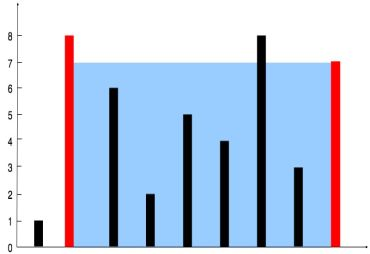## Problem: Container With Most Water

Given n non-negative integers a1, a2, …, an , where each represents a point at coordinate (i, ai). n vertical lines are drawn such that the two endpoints of line i is at (i, ai) and (i, 0)

Read More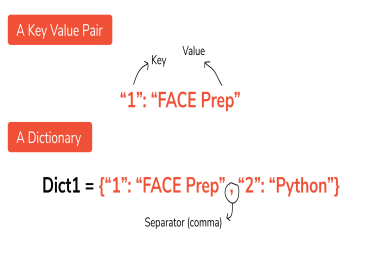## Write a function to flatten a nested dictionary separated by period

Write a function to flatten a nested dictionary separated by period

Read More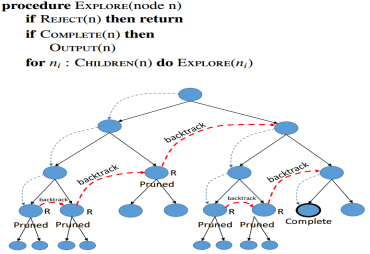## An Introduction To Backtracking

Backtracking reduces the search space since we no longer have to follow down any paths we know are invalid. This is called...

Read More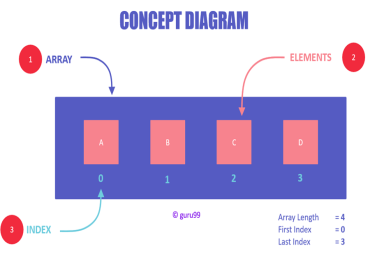## A maximal subarray

find the contiguous subarray of arr with the maximal sum of items...

Read More## Write a function that prints the least integer that is not present in a given list and cannot be represented by the summation of the sub-elements of the list

Write a function that prints the least integer that is not present in a given list and cannot be represented by the summation of the sub-elements of the list

Read More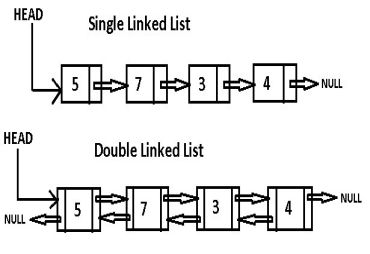## Rearrange linked list into an alternating high - low values

Given a linked list, rearrange the node values such that they appear in alternating low -> high -> low -> high ... form.

Read More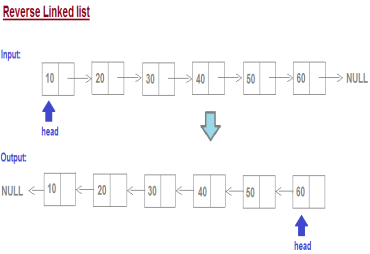## Reverse a linked list using recursion and without recursion

Reverse a linked list using recursion and without recursion. A linked list is a linear data structure where each element is a separate object. Linked list elements are not stored at the contiguous location

Read More## Given an array of integers, return a new array where each element in the new array is the number of smaller elements to the right of that element in the original input array.

Given an array of integers, return a new array where each element in the new array is the number of smaller elements to the right of that element in the original input array.

Read More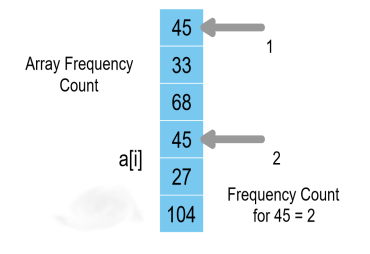## Frequency of each character in string

The problem is to print the characters along with their frequency and in the given format explained in the examples below

Read More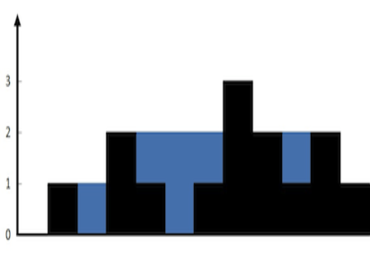## Leetcode Problem 32: Trapping Rain Water in go

Given n non-negative integers representing an elevation map where the width of each bar is 1, compute how much water it is able to trap after raining.

Read More